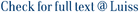A graph G = (V, E) is called a pairwise compatibility graph (PCG) if there exists a tree T, a positive edge weight function w on T, and two non-negative real numbers d(mm) &lt;= d(max), such that each leaf l(u) of T corresponds to a vertex u is an element of V and there is an edge (u, V) is an element of E if and only if d(min) &lt;= d(T,w)(l(u), l(v)) &lt;= d(max) where d(T,w)(l(u), l(v)) is the sum of the weights of the edges on the unique path from l(u), to l(v) in T. In this paper we analyze the class of PCGs in relation to two particular subclasses resulting from the cases where the constraints on the distance between the pairs of leaves concern only d(max) (LPG) or only d(min) (mLPG). In particular, we show that the union of LPG and mLPG classes does not coincide with the whole class of PCGs, their intersection is not empty, and that neither of the classes LPG and mLPG is contained in the other. Finally, we study the closure properties of the classes PCG, mLPG and LPG, under some common graph operations. In particular, we consider the following operations: adding an isolated or universal vertex, adding a pendant vertex, adding a false or a true twin, taking the complement of a graph and taking the disjoint union of two graphs. (C) 2012 Elsevier B.V. All rights reserved.

Exploring pairwise compatibility graphs / Calamoneri, Tiziana; Montefusco, Eugenio; Petreschi, Rossella; Sinaimeri, Blerina. - In: THEORETICAL COMPUTER SCIENCE. - ISSN 0304-3975. - 468:(2013), pp. 23-36. [10.1016/j.tcs.2012.11.015]

### Exploring pairwise compatibility graphs

#### Abstract

A graph G = (V, E) is called a pairwise compatibility graph (PCG) if there exists a tree T, a positive edge weight function w on T, and two non-negative real numbers d(mm) <= d(max), such that each leaf l(u) of T corresponds to a vertex u is an element of V and there is an edge (u, V) is an element of E if and only if d(min) <= d(T,w)(l(u), l(v)) <= d(max) where d(T,w)(l(u), l(v)) is the sum of the weights of the edges on the unique path from l(u), to l(v) in T. In this paper we analyze the class of PCGs in relation to two particular subclasses resulting from the cases where the constraints on the distance between the pairs of leaves concern only d(max) (LPG) or only d(min) (mLPG). In particular, we show that the union of LPG and mLPG classes does not coincide with the whole class of PCGs, their intersection is not empty, and that neither of the classes LPG and mLPG is contained in the other. Finally, we study the closure properties of the classes PCG, mLPG and LPG, under some common graph operations. In particular, we consider the following operations: adding an isolated or universal vertex, adding a pendant vertex, adding a false or a true twin, taking the complement of a graph and taking the disjoint union of two graphs. (C) 2012 Elsevier B.V. All rights reserved.
##### Scheda breve Scheda completa Scheda completa (DC)2013
disjoint union of graphs; graph complement; leaf power graphs; pcg; threshold graphs; twins
Exploring pairwise compatibility graphs / Calamoneri, Tiziana; Montefusco, Eugenio; Petreschi, Rossella; Sinaimeri, Blerina. - In: THEORETICAL COMPUTER SCIENCE. - ISSN 0304-3975. - 468:(2013), pp. 23-36. [10.1016/j.tcs.2012.11.015]
File in questo prodotto:
File
CalamoneriMontefuscoPetreschiSinaimeri_TCS2013.pdf

Solo gestori archivio

Tipologia: Versione dell'editore
Licenza: DRM (Digital rights management) non definiti
Dimensione 337.42 kB
Utilizza questo identificativo per citare o creare un link a questo documento: `https://hdl.handle.net/11385/202499`
•25
•20